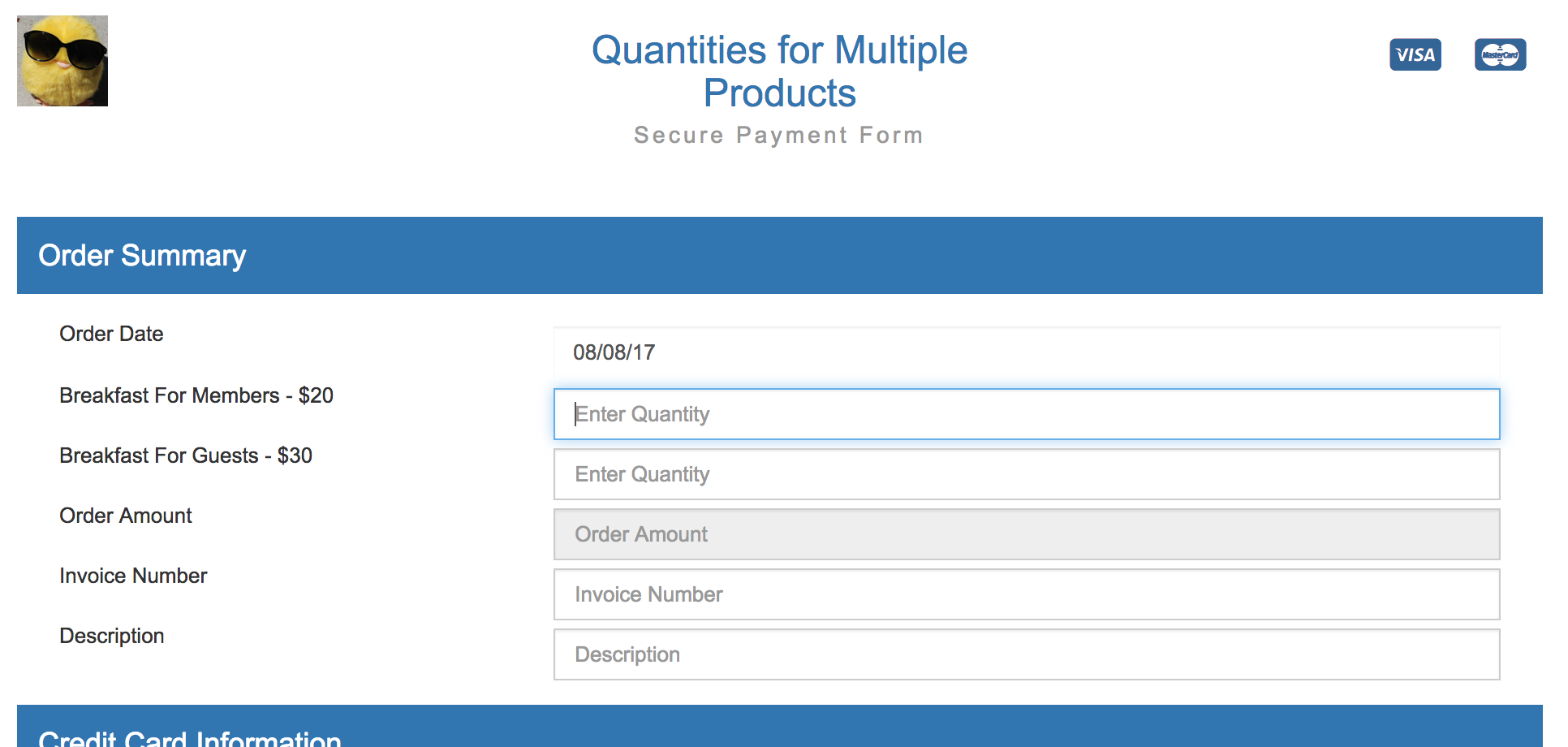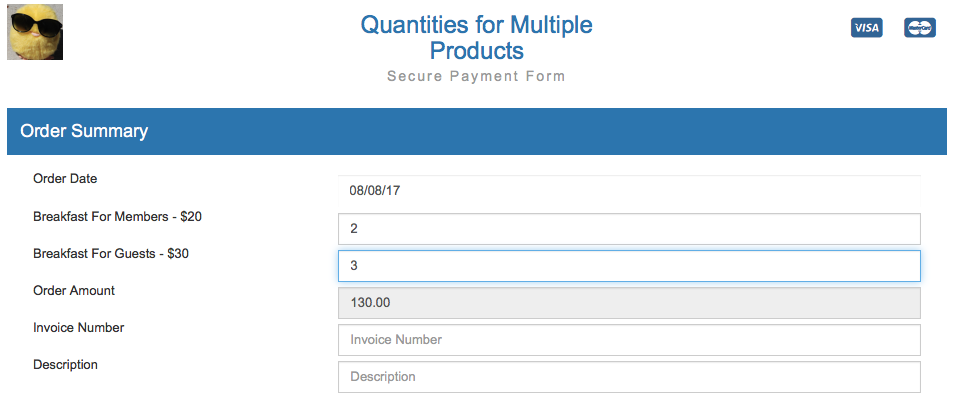Quantities for Multiple Products

This code is to add quantities for multiple products using Newtek Gateway's line items. If you choose not to use the line items, you can use Custom Fields with Custom Receipts or you can add notes about the quantities for multiple line items in the UMdescription field. The advantage to using line items is that you don't need to modify the merchant receipt and you can use Reports to see which items were bought. Once saved, the form will allow customers to enter quantities of multiple line items like the example below:When the customer enters the line items, the form will automatically total up the line items like in the example below:Please Note: The payment form does not make any changes to the product database and it does not deduct items from your inventory. If you use the product database, you will need to manually adjust the inventory and hard code the information from the product database into the payment form.

In this example we have two line items: Member Breakfast for \$20 and Guest Breakfast for \$30. We assume no modifications have been made to the payment form.

Overview

The strategy with the javascript and html is to

• Create an array of objects with each object a line item.
• Assign the same name, Line[], to all the input tags for line items so that we can create an array in the javascript for the values of all the input tags.
• Match the array of values from Line[] input tags to the quantity property for each object in the array in 1.
• Inject hidden input tags only for line items with positive line items.

The advantage to this strategy is that only one part of the javascript has to be modified to add, modify, or delete line items. But you must remember to make sure that the order of the input tags match the order of the line items in the html code. If the line items are hardcoded as hidden input tags in the form, then receipts will show every single line item even if they have 0 quantity since the form will pass UMline*quantity=0 for every line item.

Javascript

Locate this section:

<script type="text/javascript">
var submitted = false;
function submitform()
{
if(submitted) {
return false;
}
submitted=true;
return true;
}
</script>

Insert a new line directly below and add the following code (with your alterations):

<script  type="text/javascript">

//lineitem object constructor
function lineitem (productrefnum, sku, name, description, cost, quantity, taxable, hiddeninputs, subtotal)
{
this.productrefnum=productrefnum;
this.sku=sku;
this.name=name;
this.description=description;
this.cost=cost;
this.quantity=quantity;
this.taxable=taxable;
this.hiddeninputs=hiddeninputs;
this.subtotal=subtotal;

this.newhiddeninputs=newhiddeninputs;
this.newsubtotal=newsubtotal;
}

//newhiddeninputs method for lineitem object
//UMline*qty values are hidden since the form does not directly modify that variable.
function newhiddeninputs(n)
{
var hidden_inputs="";
if (this.quantity > 0)
{
hidden_inputs=hidden_inputs + "<input type=\"hidden\" name=\"UMline" + n + "productrefnum\" value=\"" + this.productrefnum + "\" />";
hidden_inputs=hidden_inputs + "<input type=\"hidden\" name=\"UMline" + n + "sku\" value=\"" + this.sku + "\" />";
hidden_inputs = hidden_inputs + "<input type=\"hidden\" name=\"UMline" + n + "name\" value=\"" + this.name + "\" />";
hidden_inputs = hidden_inputs + "<input type=\"hidden\" name=\"UMline" + n + "description\" value=\"" + this.description + "\" />";
hidden_inputs = hidden_inputs + "<input type=\"hidden\" name=\"UMline" + n + "cost\" value=\"" + this.cost + "\" />";
hidden_inputs = hidden_inputs + "<input type=\"hidden\" name=\"UMline" + n + "qty\" value=\"" + this.quantity + "\" />";
hidden_inputs = hidden_inputs + "<input type=\"hidden\" name=\"UMline" + n + "taxable\" value=\"" + this.taxable + "\" />";
this.hiddeninputs=hidden_inputs;
}
}

//newsubtotal method for lineitem object
function newsubtotal()
{
this.subtotal=this.quantity*(this.cost*1.0);
}

//main function to update the amount. We match quantities of all the line items to
//the products. Then we change the hidden inputs using a
//<div id="hiddenvariables>&nbsp;</div> tag only if the quantity > 0 for the product.
function updateAmount()
{

//we pull all the elements of the form which are named "Line[]" so that we'll be able to
//cycle through the assigning product quantities with a for loop based on the number of
//input fields for the products on the form. Without the [] notation
//we'd have to have a separate declaration line for each product.quantity
var myForm = document.forms.epayform;
var lineItems = myForm.elements["Line[]"];

//assign quantities to lineqty
var lineqty = new Array(lineItems.length); //lineqty size needs to be explicitly defined
for (var q = 0;  q < lineItems.length; q++) //must use < since arrays start index at 0
{
lineqty[q] = lineItems[q].value;
}

//create lineitem objects for each product.
//To ease future maintenance of code we use an array to house the objects
//It's possible we may not need every single property of the product members to be declared
//but it's done just to avoid any possible errors with undefined properties.
//It's merchant's choice how many properties they really want to pass through on their
//transaction details and/or receipts.
//We initialize .quantity=0, .subtotal=0, .hiddeninputs="".
var products = new Array();

products = new lineitem();
products.productrefnum = "57"
products.sku = "breakfastmembers";
products.name = "Breakfast for Members";
products.description = "Breakfast for members";
products.cost = 20.00;
products.quantity = 0;
products.taxable = "N";
products.subtotal = 0;
products.hiddeninputs = "";

products = new lineitem();
products.productrefnum = "59"
products.sku = "breakfastnonmembers";
products.name = "Breakfast for Non-Members";
products.description = "Breakfast for non-members";
products.cost = 30.00;
products.quantity = 0;
products.taxable = "N";
products.subtotal = 0;
products.hiddeninputs = "";

//assign quantities to the product objects
for (var l=1; l < lineqty.length; l++)  //must use < since arrays start index at 0
{
if (lineqty.length > 0)
{
products[l].quantity = lineqty[l];
}
}

//calculate subtotals
var total=0;
for (var t=1; t < products.length; t++)  //must use < since arrays start index at 0
{
if (products[t].quantity > 0)
{
products[t].newsubtotal();
total=total + products[t].subtotal;
}
}

//create hidden inputs for form
var hiddeninputscomplete= "";
for (var h=1; h < products.length; h++)  //must use < since arrays start index at 0
{
if (products[h].quantity > 0)
{
products[h].newhiddeninputs(h);
hiddeninputscomplete = hiddeninputscomplete + products[h].hiddeninputs;
document.getElementById('hiddenvariables').innerHTML = hiddeninputscomplete;
}
}

//assign total to UMamount and modify form to send the amount to the gateway.
total = Math.round((total*1)*100)/100;
document.epayform.UMamount.value = total;
document.getElementByName('UMamount').innerHTML = total;

}
function CurrencyFormatted(amount)
{
var i = parseFloat(amount);
if(isNaN(i)) { i = 0.00; }
var minus = '';
if(i < 0) { minus = '-'; }
i = Math.abs(i);
i = parseInt((i + .005) * 100);
i = i / 100;
s = new String(i);
if(s.indexOf('.') < 0) { s += '.00'; }
if(s.indexOf('.') == (s.length - 2)) { s += '0'; }
s = minus + s;
return s;
}
</script>

HTML

After you have completed entering the javascript, you will need to make these changes to the HTML. Find the part of the form with the hidden tags, which should look something like this:

<input type="hidden" name="UMsubmit" value="1">
<input type="hidden" name="UMkey" value="[UMkey]">
<input type="hidden" name="UMredirDeclined" value="[UMredirDeclined]">
<input type="hidden" name="UMredirApproved" value="[UMredirApproved]">
<input type="hidden" name="UMhash" value="[UMhash]">
<input type="hidden" name="UMcommand" value="[UMcommand]">
<input type="hidden" name="UMtax" value="[UMtax]">
<input type="hidden" name="UMinvoice" value="[UMinvoice]">
<input type="hidden" name="UMcustid" value="[UMcustid]">
<input type="hidden" name="UMrecurring" value="[UMrecurring]">
<input type="hidden" name="UMbillamount" value="[UMbillamount]">
<input type="hidden" name="UMcustreceipt" value="yes">
<input type="hidden" name="UMschedule" value="[UMschedule]">
<input type="hidden" name="UMnumleft" value="[UMnumleft]">
<input type="hidden" name="UMstart" value="[UMstart]">
<input type="hidden" name="UMexpire" value="[UMexpire]">
<input type="hidden" name="UMdescription" value="[UMdescription]">
<input type="hidden" name="UMechofields" value="[UMechofields]">
<input type="hidden" name="UMformString" value="[UMformString]">

First, remove this line if it appears:

<input type="hidden" name="UMamount" value="[UMamount]">

Then, add this line right below the rest of the hidden values:

<div id="hiddenvariables">&nbsp;</div>

Find where you want to add the line items. Most people add it between the Order Date row and the Amount Row. Place the following code:

<div class="form-group">
<span class="col-form-label col-sm-4 col-xs-12 "></span>
<div class="col-md-8 col-xs-12 ">
<input class="form-control" type="hidden" value="[UMamount]" name="Line[]" value="">
</div>
</div>
<div class="form-group">
<span class="col-form-label col-sm-4 col-xs-12 ">Breakfast For Members - \$20</span>
<div class="col-md-8 col-xs-12 ">
<input class="form-control" type="text" name="Line[]" value="" placeholder="Enter Quantity" onChange="updateAmount()">
</div>
</div>
<div class="form-group">
<span class="col-form-label col-sm-4 col-xs-12 ">Breakfast For Guests - \$30</span>
<div class="col-md-8 col-xs-12 ">
<input class="form-control" type="text" name="Line[]" value="" placeholder="Enter Quantity" onChange="updateAmount()">
</div>
</div>

Find the spot in the form where the Amount is displayed to the customer. If you search for [UMamount] and find the second occurrence, it should look like this:

<div class="form-group">
<span class="col-form-label col-sm-4 col-xs-12 ">Order Amount</span>
<div class="col-md-8 col-xs-12 ">